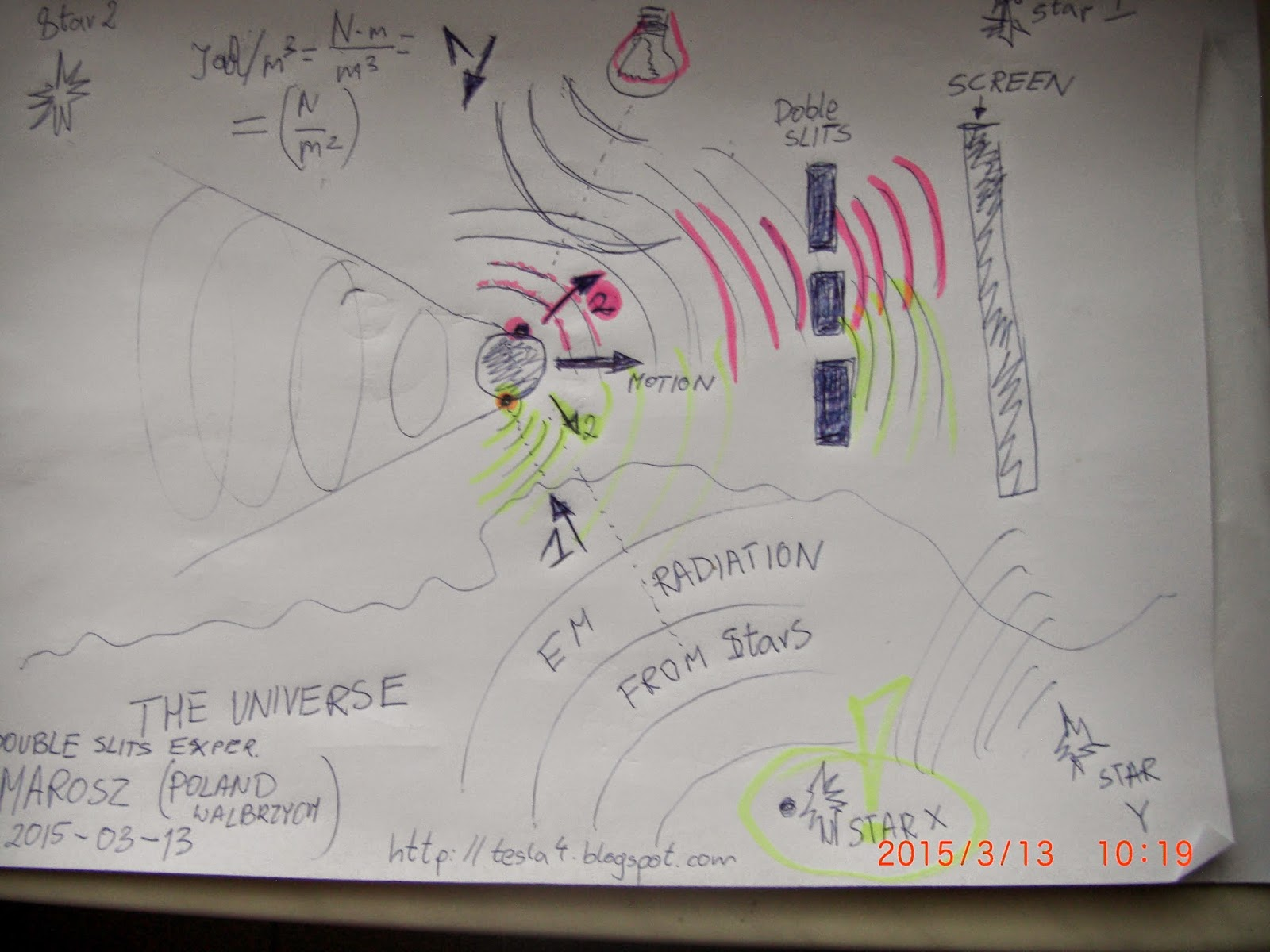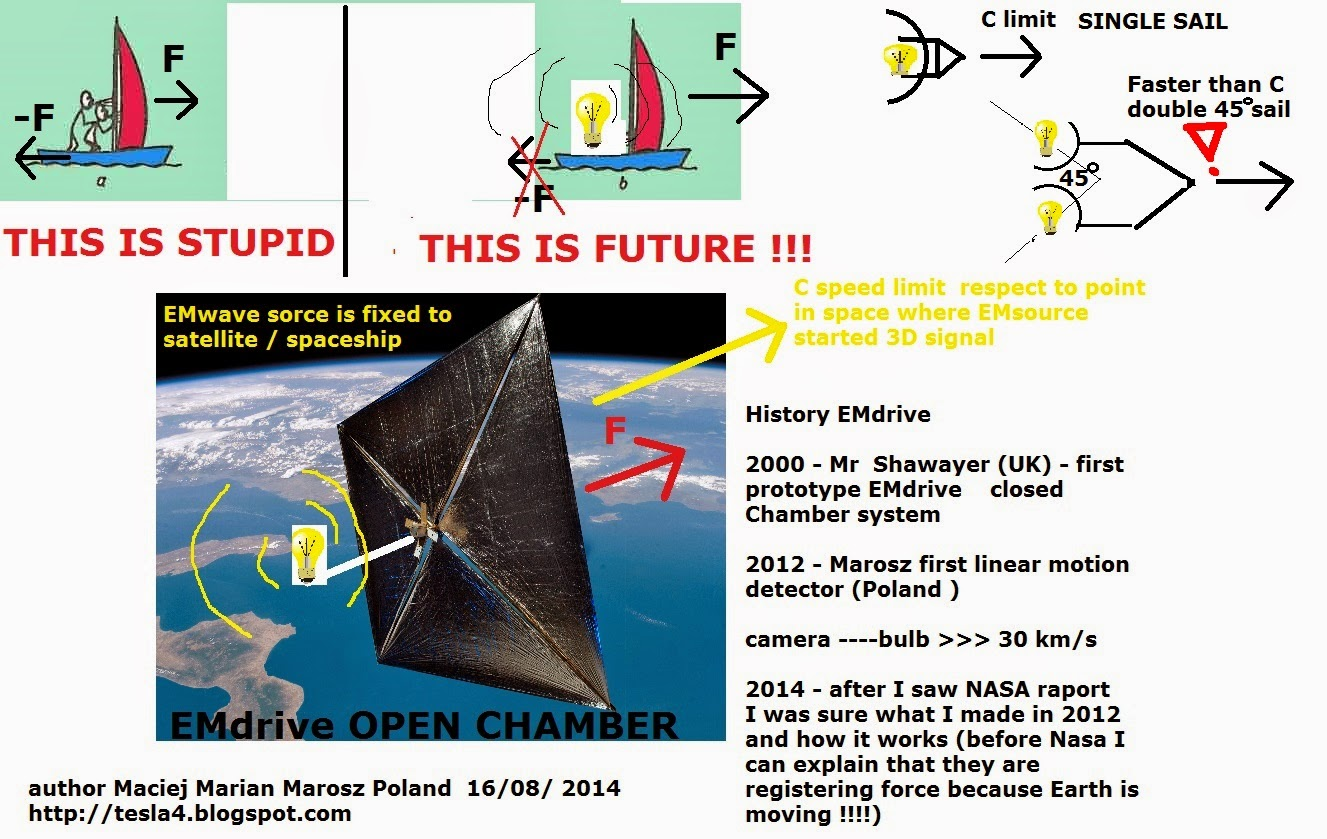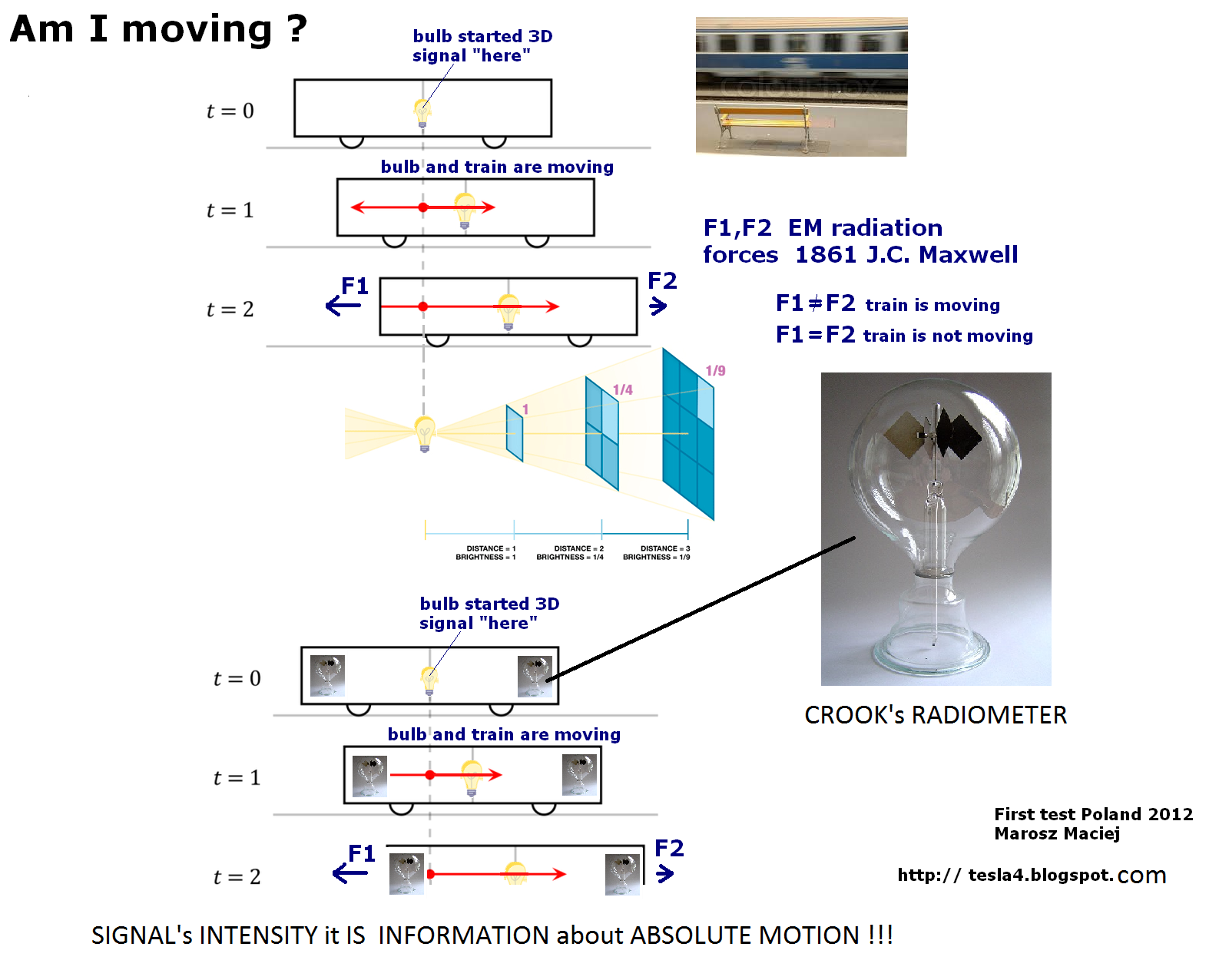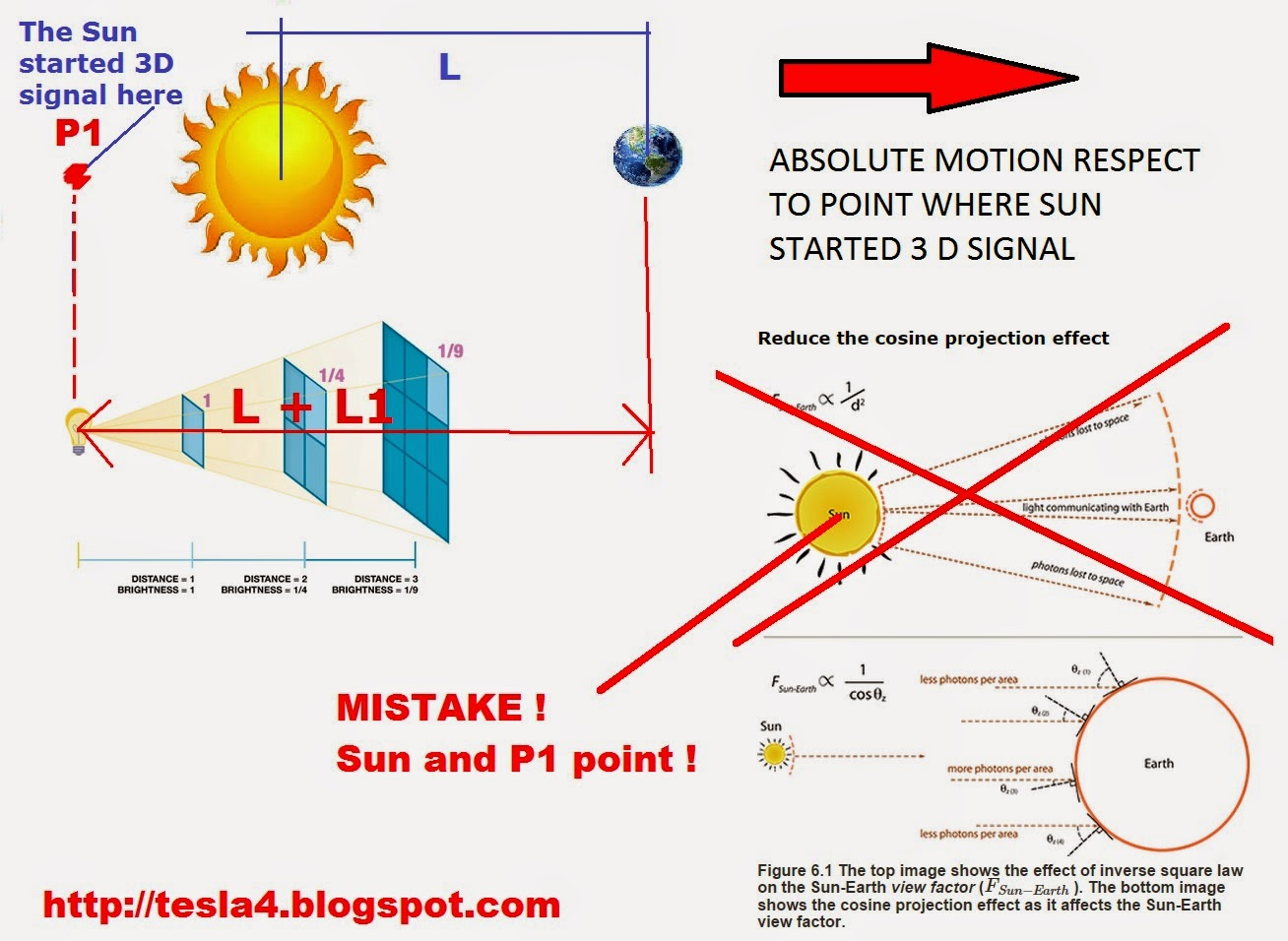Simplicity as a Primary Condition of Nature and Evolution.
=.
The evolution of Nature is going from simple to complex therefore
I will take the simplest physical parameters in order to explain
the primary conditions of evolution in Nature.
1.
The simplest reference frame is two dimensions (2D).
There are two kinds of 2D: Euclidian (relative) and
Pseudo-Euclidian (absolute according to SRT).
What Euclidian 2D is - everybody knows.
What Pseudo-Euclidian (negative - 2D) is - nobody knows.
In my opinion (- 2D) is Zero Vacuum reference frame: T= - 273,15 . . . . .
2.
In this simplest negative reference frame (- 2D) only flat - circle
particles can exist: c/d = 3,14 . . . . and they are the simplest original /
primary quantum particles of Nature.
3.
These quantum particles in their simplicity contain their own
inner – natural power / energy and impulses: h and h*=h/2pi.
4.
These particles obey "the law of conservation and transformation energy".
This law is not book-keeper's calculations of "debit – credit".
This law means:
the simplest particles can keep and somehow transform their energy.
=.
Only on these simplest physical parameters (T= - 273,15 . . , c/d = 3,14 . . . ,
h and h*=h/2pi ) and "the law of conservation and transformation energy"
can be constructed the reliable castle for Quantum theory.
==..
More than 300 years ago Newton wrote:
" For the basic problem of philosophy seems to be to discover
the forces of nature from the phenomena of motions
and then to demonstrate the other phenomena from these forces."
This Newton's offer is carried out fully in Quantum physics.
==...
From the state of Simplicity was created and evolved
the complex World of Matter, Consciousness, Knowledge.
==..
Best wishes.
==..
QUANTUM ? IT IS IDIOTISM^2THEY BLOCKING REAL SICENCE ( if I don't know I'm using QUANTUM ? )

EM -drive future

They speak about Qunatum's space fluctuation

in my opinion below EM drive rule is more simple :

VERY IMPORTANT ! fact for each my TEST

James Clerk Maxwell, in 1861–64, published his theory of electromagnetic fields and radiation, which shows that light has momentum and thus can exert pressure on objects.

Radiation pressure in classical electromagnetism:
... it was published by James Clerk Maxwell in 1862
,and proven experimentally ( 1900 - 1901)
> http://en.wikipedia.org/wiki/Solar_sailAM I MOVING ? Each body is moving respeact to point where was in past !!!WHY WE MUST USE Point p1 ? Below typical mistake in books ( physics- optica ) LEFT side is corect Right side ????SUN WAS IN PAST IN POINT P1
WHEN I LOOKING ON STAR I NEVER SEE HER ACTUAL "fresh" position !!!

GRAVITATION AND MOTION ?

m....................M.......................m >>> motion

where mass M started gravitation ?

Mass m left and m right are registering the same intensity ?
what about natural fall Law ?Somebody wrote : 1-D is simpler than 2-D.
The 1-D figure is explained by "theory of string-particle".
The result is written in the book "The trouble with Physics" by Lee Smolin.

#
Other wrote: a triangle is simpler than circle.
The triangle has angles.
To create angles needs some kind of forces.
Without forces every flat geometrical figure would change into circle.
==..
Originally Posted By: socratus
Somebody wrote : 1-D is simpler than 2-D.
The 1-D figure is explained by "theory of string-particle".
The result is written in the book "The trouble with Physics" by Lee Smolin.

#
Other wrote: a triangle is simpler than circle.
The triangle has angles.
To create angles needs some kind of forces.
Without forces every flat geometrical figure would change into circle.
==..

More details.

Somebody wrote : 1-D is simpler than 2-D.
The 1-D ( line with Planck's length but without thickness) is explained
by "theory of string-particle". Theorists try to understand 1-D string in 11-D.
The result is written in the book "The trouble with Physics" by Lee Smolin.
In the others words:
Where is Alice?
Alice is in the 1-D String, at 11-D Wonderland.

#
Other wrote: a triangle is simpler than circle.
The argument.
To make a triangle is needed to apply force at three different locations.
To make a circle is needed to apply force at all locations.
Therefore " a triangle is simpler than circle."
The refutation.
To apply " force at all locations " means that the particle
is in an equilibrium state . . . . in relax state . . . in potential state.
The equilibrium is primary state of particle.
To change equilibrium needs forces.
For example:
To create line needs forces in two different directions.
To create triangle needs forces in three different directions. . . . etc
Without forces every flat particle will change into a symmetrical
equilibrium - circle state: c/d=pi=3,14 . . . .
==….